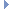OpenMM
AmoebaStretchBendForce Class Reference

This class implements the Amoeba stretch-bend interaction. More...Inheritance diagram for AmoebaStretchBendForce:

List of all members.

## Public Member Functions

def getNumStretchBends
getNumStretchBends(self) -> int
addStretchBend(self, particle1, particle2, particle3, lengthAB, lengthCB, angle, k1, k2) -> int
def getStretchBendParameters
Get the force field parameters for a stretch-bend term.
def setStretchBendParameters
Set the force field parameters for a stretch-bend term.
def updateParametersInContext
Update the per-stretch-bend term parameters in a Context to match those stored in this Force object.
def usesPeriodicBoundaryConditions
usesPeriodicBoundaryConditions(self) -> bool
def __init__
__init__(self) -> AmoebaStretchBendForce __init__(self, other) -> AmoebaStretchBendForce

this

## Detailed Description

This class implements the Amoeba stretch-bend interaction.

To use it, create a StretchBendForce object then call addStretchBend() once for each stretch-bend. After a stretch-bend has been added, you can modify its force field parameters by calling setStretchBendParameters(). This will have no effect on Contexts that already exist unless you call updateParametersInContext().

## Constructor & Destructor Documentation

 def __init__ ( self, args )

__init__(self) -> AmoebaStretchBendForce __init__(self, other) -> AmoebaStretchBendForce

Create an AmoebaStretchBendForce.

## Member Function Documentation

 def addStretchBend ( self, particle1, particle2, particle3, lengthAB, lengthCB, angle, k1, k2 )

addStretchBend(self, particle1, particle2, particle3, lengthAB, lengthCB, angle, k1, k2) -> int

Add a stretch-bend term to the force field.

Parameters:
 particle1 (int) the index of the first particle connected by the stretch-bend particle2 (int) the index of the second particle connected by the stretch-bend particle3 (int) the index of the third particle connected by the stretch-bend lengthAB (double) the equilibrium length of the stretch-bend in bond ab [particle1, particle2], measured in nm lengthCB (double) the equilibrium length of the stretch-bend in bond cb [particle3, particle2], measured in nm angle (double) the equilibrium angle in radians k1 (double) the force constant of the product of bond ab and angle a-b-c k2 (double) the force constant of the product of bond bc and angle a-b-c (optional, default is the same as k1)
Returns:
(int) the index of the stretch-bend that was added
 def getNumStretchBends ( self )

getNumStretchBends(self) -> int

Get the number of stretch-bend terms in the potential function

 def getStretchBendParameters ( self, index )

Get the force field parameters for a stretch-bend term.

Parameters:
 index (int) the index of the stretch-bend for which to get parameters
Returns:
(int) the index of the first particle connected by the stretch-bend
(int) the index of the second particle connected by the stretch-bend
(int) the index of the third particle connected by the stretch-bend
(double) the equilibrium length of the stretch-bend in bond ab [particle1, particle2], measured in nm
(double) the equilibrium length of the stretch-bend in bond cb [particle3, particle2], measured in nm
(double) the equilibrium angle in radians
(double) the force constant of the product of bond ab and angle a-b-c
(double) the force constant of the product of bond bc and angle a-b-c
 def setStretchBendParameters ( self, index, particle1, particle2, particle3, lengthAB, lengthCB, angle, k1, k2 )

Set the force field parameters for a stretch-bend term.

Parameters:
 index (int) the index of the stretch-bend for which to set parameters particle1 (int) the index of the first particle connected by the stretch-bend particle2 (int) the index of the second particle connected by the stretch-bend particle3 (int) the index of the third particle connected by the stretch-bend lengthAB (double) the equilibrium length of the stretch-bend in bond ab [particle1, particle2], measured in nm lengthCB (double) the equilibrium length of the stretch-bend in bond cb [particle3, particle2], measured in nm angle (double) the equilibrium angle in radians k1 (double) the force constant of the product of bond ab and angle a-b-c k2 (double) the force constant of the product of bond bc and angle a-b-c (optional, default is the same as k1)
 def updateParametersInContext ( self, context )

Update the per-stretch-bend term parameters in a Context to match those stored in this Force object.

This method provides an efficient method to update certain parameters in an existing Context without needing to reinitialize it. Simply call setStretchBendParameters() to modify this object's parameters, then call updateParametersInContext() to copy them over to the Context.

The only information this method updates is the values of per-stretch-bend term parameters. The set of particles involved in a term cannot be changed, nor can new terms be added.

 def usesPeriodicBoundaryConditions ( self )

usesPeriodicBoundaryConditions(self) -> bool

Returns whether or not this force makes use of periodic boundary conditions.

Returns:
(bool) true if nonbondedMethod uses PBC and false otherwise

Reimplemented from Force.

## Member Data Documentation

Reimplemented from Force.

The documentation for this class was generated from the following file:
• openmm.py